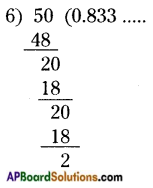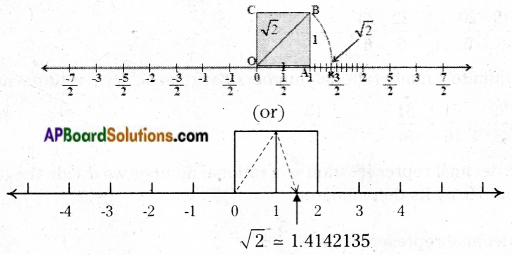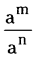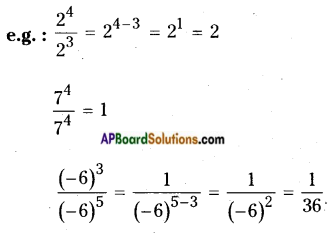# AP Board 9th Class Maths Notes Chapter 1 Real Numbers

Students can go through AP Board 9th Class Maths Notes Chapter 1 Real Numbers to understand and remember the concepts easily.

## AP State Board Syllabus 9th Class Maths Notes Chapter 1 Real Numbers

→ Numbers of the form $$\frac{p}{q}$$ where p and q are integers and q ≠ 0 are called rational numbers, represented by ‘Q’.

→ There are infinitely many rational numbers between any two integers.
E.g.: 3 < $$\frac{19}{6}$$, $$\frac{20}{6}$$, $$\frac{21}{6}$$, $$\frac{22}{6}$$, $$\frac{23}{6}$$, ……. < 4

→ There are infinitely many rational numbers between any two rational numbers.
E.g.: $$\frac{3}{4}$$ < $$\frac{29}{8}$$ < $$\frac{71}{16}$$ < $$\frac{81}{14}$$ ……. < $$\frac{13}{2}$$

→ To find the decimal representation of a rational number we divide the numerator of a rational number by its denominator.
E.g.: The decimal representation of $$\frac{5}{6}$$ is∴ $$\frac{5}{6}$$ = 0.833 …. = 0.8 $$\overline{3}$$→ Every rational number can be expressed as a terminating decimal or as a non-terminating repeating decimal. Conversely every terminating decimal or non¬terminating recurring decimal can be expressed as a rational number.
E.g.: 1.6 $$\overline{2}$$ = $$\frac{161}{99}$$

→ A rational number whose denominator consists of only 2’s or 5’s or a combination of 2’s and 5’s can be expressed as a terminating decimal.
E.g. : $$\frac{13}{32}$$ can be expressed as a terminating decimal (∵ 32 = 2 × 2 × 2 × 2 × 2)
$$\frac{7}{125}$$ can be expressed as a terminating decimal (∵ 125 = 5 × 5 × 5)
$$\frac{24}{40}$$ can be expressed as a terminating decimal (∵ 40 = 2 × 2 × 2 × 5)

→ Numbers which can’t written in the form $$\frac{p}{q}$$ where p and q are integers and q ≠ 0, are called irrational numbers.
E.g.: √2, √3, √5,….. etc.
The decimal form of an irrational number is neither terminating nor recurring decimal.

→ Irrational numbers can be represented on a number line using Pythagoras theorem.
E.g.: Represent √2 on a number line.→ If ‘n’ is a natural number which is not a perfect square, then √n is always an irrational number.
E.g.: 2, 3, 5, 7, 8, …… etc., are not perfect squares.
∴ √2, √3, √5, √7 and √8 are irrational numbers.→ We often write π as $$\frac{22}{7}$$ there by π seems to be a rational number; but π is not a rational number.

→ The collection of rational numbers together with irrational numbers is called set of Real numbers.
R = Q ∪ S

→ If a and b are two positive rational numbers such that ab is not a perfect square, then , √ab is an irrational number between ‘a’ and b’.
E.g.: Consider any two rational numbers 7 and 4.
7 × 4 = 28 is not a perfect square; then √28 lies between 4 and 7.
i.e., 4 < √28 < 1

→ If ‘a’ is a rational number and ‘b’ is arty irrational number then a + b, a – b, a.b or $$\frac{a}{b}$$ is an irrational number.
E.g.: Consider 7 and √5 then 7 + √5, 7 – √5, 7√5 and $$\frac{7}{\sqrt{5}}$$= are all irrational numbers.

→ If the product of any two irrational numbers is a rational number, then they are said to be the rationalising factor of each other.
E.g.: Consider any two irrational number 7√3 and 5√3.
7√3 × 5√3 = 7 × 5 × 3 = 105 a rational number.
Also 7√3 × √3 = 21 – a rational number.
5√3 × √3 = 15 – a rational number.
So the rationalising factor of an irrational number is not unique.

→ The general form of rationalising factor (R.F.) of (a ± √b} is (a ∓ √b). They are called conjugates to each other.

→ Laws of exponents:

i) am × an = am+n
e.g.: 54 . 5-3 = 54+(-3) = 51 = 5

ii) (am)n = amn
e.g.: (43)2 = 43×2 = 46

iii)= am-n if (m > n)
= 1 if m = n
= $$\frac{1}{a^{n-m}}$$ if (m < n)iv) am . bm = (ab)m
e.g.:(-5)3 . (2)3 = (-5 × 2)3 =(-10)3

v) $$\frac{1}{a^{n}}$$ = a-n
e.g.: (6)-3 = $$\frac{1}{6^{3}}$$ = $$\frac{1}{216}$$

vi) a0 = 1
e.g.: $$\left(\frac{-3}{4}\right)^{0}$$ = 1
Where a, b are rationals and m, n are integers.

→ Let a, b be any two rational numbers such that a = bn then b = $$\sqrt[n]{a}$$ = $$(\mathrm{a})^{1 / \mathrm{n}}$$
Here ‘b’ is called nth root of a.
e.g.: 42 = 16 then $$16^{1 / 2}$$ or $$\sqrt{16}$$
34 = 81 then 3 = $$\sqrt{81}$$ or $$(81)^{1 / 4}$$

→ Let ‘a’ be a positive number and n > 1 then $$\sqrt[n]{a}$$ i.e., nth root of a is called a surd.